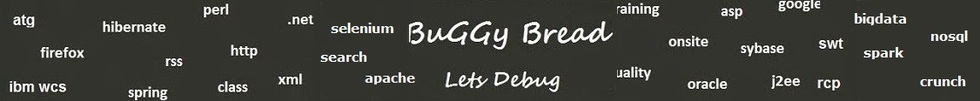## Search Java Classes and Packages

Search Java Frameworks and Libraries

255581 classes and counting ...
 Search Tips Index Status

#Org.moeaframework.core Classes and Interfaces - 81 results found.
 Name Description Type Package Framework AdaptiveMetropolis The adaptive metropolis (AM) operator. Class org.moeaframework.core.operator MOEA AdaptiveMultimethodVariation Auto-adaptive multi-method recombination operator. Class org.moeaframework.core MOEA AdditiveEpsilonIndicator Class org.moeaframework.core MOEA AdditiveEpsilonIndicatorFitnessEvaluator Class org.moeaframework.core MOEA AggregateConstraintComparator Compares solutions based on their magnitude of constraint violations. Class org.moeaframework.core MOEA AlgorithmFactory Factory for creating algorithm instances. Class org.moeaframework.core MOEA AlgorithmProvider Defines an SPI for algorithms. Class org.moeaframework.core MOEA BinaryIntegerVariable Decision variable for integers encoded as a binary string. Class org.moeaframework.core MOEA BinaryVariable Decision variable for binary strings. Class org.moeaframework.core MOEA BitFlip Bit flip mutation operator. Class org.moeaframework.core.operator MOEA ChainedComparator Applies any number of comparators in succession, returning the result from the first comparator producing a non-zero return value. Class org.moeaframework.core MOEA CompoundVariation Construct a variation operator applying one or more variations sequentially. Class org.moeaframework.core MOEA Contribution Measures the contribution of the approximation set to the reference set. Class org.moeaframework.core MOEA CrowdingComparator Compares solutions using their crowding distance. Class org.moeaframework.core MOEA CrowdingDistanceFitnessEvaluator Class org.moeaframework.core MOEA DifferentialEvolution Differential evolution (DE) variation operator. Class org.moeaframework.core.operator MOEA DifferentialEvolutionSelection Selection operator to be used in conjunction with the DifferentialEvolution operator. Class org.moeaframework.core.operator MOEA DominanceComparator Interface for comparing two solutions using a dominance relation. Interface org.moeaframework.core MOEA EncodingUtils Helper methods for working with various decision variable types and encodings. Class org.moeaframework.core MOEA EpsilonBoxDominanceComparator Class org.moeaframework.core MOEA EpsilonBoxObjectiveComparator Class org.moeaframework.core MOEA FitnessBasedArchive Maintains a non-dominated archive of solutions with a maximum capacity. Class org.moeaframework.core MOEA FitnessComparator Compares two solutions based on their FITNESS_ATTRIBUTE value. Class org.moeaframework.core MOEA GAVariation Operator for applying a crossover and mutation operation in succession. Class org.moeaframework.core MOEA GenerationalDistance Generational distance indicator. Class org.moeaframework.core MOEA Grammar Decision variable for grammars. Class org.moeaframework.core MOEA GrammarCrossover Single-point crossover for grammars. Class org.moeaframework.core.operator MOEA GrammarMutation Uniform mutation for grammars. Class org.moeaframework.core.operator MOEA HUX Half-uniform crossover (HUX) operator. Class org.moeaframework.core.operator MOEA Hypervolume Hypervolume indicator. Class org.moeaframework.core MOEA HypervolumeContributionFitnessEvaluator Class org.moeaframework.core MOEA HypervolumeFitnessEvaluator Indicator-based fitness using the hypervolume metric. Class org.moeaframework.core MOEA IndicatorFitnessEvaluator Abstract class for assigning fitnesses based on an indicator. Class org.moeaframework.core MOEA IndicatorUtils Collection of methods shared by indicators. Class org.moeaframework.core MOEA InjectedInitialization Initialization method that injects pre-defined solutions into the initial population. Class org.moeaframework.core MOEA Insertion Insertion mutation operator. Class org.moeaframework.core.operator MOEA InvertedGenerationalDistance Inverted generational distance indicator. Class org.moeaframework.core MOEA LexicographicalComparator Compares solutions lexicographically. Class org.moeaframework.core MOEA MaximumParetoFrontError Maximum Pareto front error metric. Class org.moeaframework.core MOEA NondominatedSortingComparator Compares two solutions using their rank and crowding distance. Class org.moeaframework.core MOEA NormalizedIndicator Class org.moeaframework.core MOEA Normalizer Normalizes populations so that all objectives reside in the range [0, 1]. Class org.moeaframework.core MOEA ObjectiveComparator Compares two solutions using the value of a specific objective. Class org.moeaframework.core MOEA OnePointCrossover One-point or single-point crossover. Class org.moeaframework.core MOEA OperatorFactory Factory for creating and variation (e. Class org.moeaframework.core MOEA OperatorProvider Defines an SPI for initializing different operators. Class org.moeaframework.core MOEA ParetoConstraintComparator Compares solutions based on the Pareto efficiency of their constraints. Class org.moeaframework.core MOEA ParetoDominanceComparator Compares two solutions using aggregate constraint violation and the Pareto dominance relation as originally proposed by Kalyanmoy Deb. Class org.moeaframework.core MOEA ParetoObjectiveComparator Compares two solutions using the Pareto dominance relation on the objectives. Class org.moeaframework.core MOEA PCX Parent-centric crossover (PCX) operator. Class org.moeaframework.core.operator MOEA Permutation Decision variable for permutations. Class org.moeaframework.core MOEA PM Polynomial mutation (PM) operator. Class org.moeaframework.core.operator MOEA PMX Partially mapped crossover (PMX) operator. Class org.moeaframework.core.operator MOEA PointMutation Mutates a program by randomly selecting nodes in the expression tree and replacing the node with a new, compatible, randomly-selected node. Class org.moeaframework.core.operator MOEA ProblemFactory Factory for creating optimization problem instances and their corresponding reference sets, if known. Class org.moeaframework.core MOEA ProblemProvider Defines an SPI for instantiation optimization problems. Class org.moeaframework.core MOEA Program A decision variable for programs. Class org.moeaframework.core MOEA ProviderNotFoundException Exception indicating a provider was not found. Class org.moeaframework.core MOEA QualityIndicator Evaluates multiple indicators while avoiding repetitive computations. Class org.moeaframework.core MOEA R1Indicator Computes the R1 indicator. Class org.moeaframework.core MOEA R2Indicator Computes the R2 indicator. Class org.moeaframework.core MOEA R3Indicator Computes the R3 indicator. Class org.moeaframework.core MOEA RandomInitialization Initializes all built-in decision variables randomly. Class org.moeaframework.core MOEA RankComparator Compares solutions using their RANK_ATTRIBUTE value. Class org.moeaframework.core MOEA RealVariable Decision variable for real values. Class org.moeaframework.core MOEA RIndicator Abstract class for implementing R indicator functions. Class org.moeaframework.core MOEA RIndicator .ChebychevUtility Chebychev (also referred to as Tchebycheff) utility function. Class org.moeaframework.core MOEA RIndicator .LinearWeightedSumUtility Utility computed as the sum of the weighted objective values. Class org.moeaframework.core MOEA RIndicator .UtilityFunction assume the solutions have been normalized. Interface org.moeaframework.core MOEA SBX Simulated binary crossover (SBX) operator. Class org.moeaframework.core.operator MOEA Spacing Spacing metric. Class org.moeaframework.core MOEA SPX Simplex crossover (SPX) operator. Class org.moeaframework.core.operator MOEA StandardOperators Default provider of operators. Class org.moeaframework.core MOEA SubtreeCrossover Exchanges a randomly-selected subtree from one program with a compatible, randomly-selected subtree from another program. Class org.moeaframework.core.operator MOEA Swap Swap mutation operator. Class org.moeaframework.core.operator MOEA TournamentSelection Deterministic tournament selection operator. Class org.moeaframework.core MOEA TwoPointCrossover Two-point crossover. Class org.moeaframework.core MOEA UM Uniform mutation (UM) operator. Class org.moeaframework.core.operator MOEA UNDX Unimodal Normal Distribution Crossover (UNDX) operator. Class org.moeaframework.core.operator MOEA UniformCrossover Crossover operator where each index is swapped with a specified probability. Class org.moeaframework.core MOEA UniformSelection Uniform selection operator. Class org.moeaframework.core MOEA